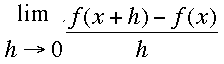SEARCH HOMEMath Central Quandaries & QueriesQuestion from Sheila, a student: hi! our teacher gave us this question as a challenge and even he couldn't figure it out: Differentiate x^(1/3) [aka the cube root of x] using first principles. i know the answer is 1/(3.x^2/3), but how is it possible using first principles? thank you, SheilaHi Sheila,

If f(x) = x1/3 then you need to evaluateand the numerator is

(x + h)1/3 - x1/3

Let a = (x + h)1/3 and b = x1/3 and recall the difference of cubes

a3 - b3 = (a - b)(a2 + ab + b2)

Multiply the numerator and denominator inside the limit by (a2 + ab + b2) and the numerator becomes

a3 - b3 = (x + h) - x = h

and the denominator becomes

h[(x+h)2/3 + x1/3 (x + h)1/3 + x2/3]

The numerator and denominator each have a factor of h which will cancel and then you can take the limit as h approaches zero.

I hope this helps,
HarleyMath Central is supported by the University of Regina and The Pacific Institute for the Mathematical Sciences.